# RD Sharma Class 8 Solutions Chapter 3 Squares and Square Roots Ex 3.9

In this chapter, we provide RD Sharma Class 8 Solutions Chapter 3 Squares and Square Roots Ex 3.9 for English medium students, Which will very helpful for every student in their exams. Students can download the latest RD Sharma Class 8 Solutions Chapter 3 Squares and Square Roots Ex 3.9 Maths pdf, free RD Sharma Class 8 Solutions Chapter 3 Squares and Square Roots Ex 3.9 Maths book pdf download. Now you will get step by step solution to each question.

### RD Sharma Solutions for Class 8 Chapter 3 Squares and Square Roots Ex 3.9 Download PDF

Using square root table, find the square roots of the following :

Question 1.
7
Solution: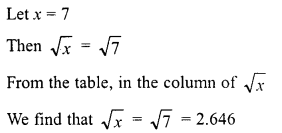Question 2.
15
Solution:Question 3.
74
Solution:Question 4.
82
Solution:Question 5.
198
Solution:Question 6.
540
Solution:Question 7.
8700
Solution:Question 8.
3509
Solution: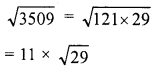Question 9.
6929
Solution:Question 10.
25725
Solution: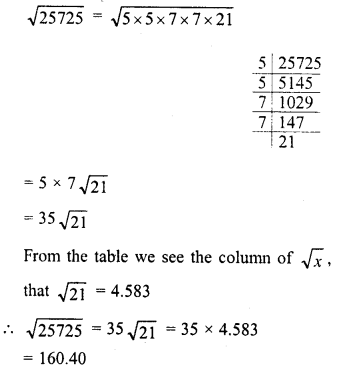Question 11.
1312
Solution:Question 12.
4192
Solution:Question 13.
4955
Solution:Question 14.
99144
Solution:Question 15.
57169
Solution: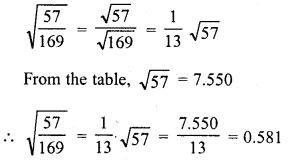Question 16.
101169
Solution:Question 17.
13.21
Solution: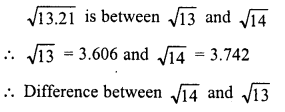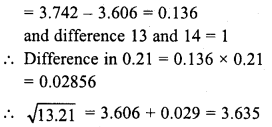Question 18.
21.97
Solution: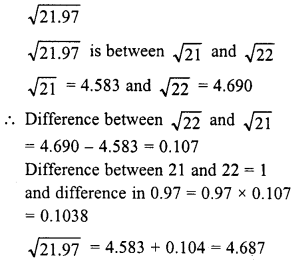Question 19.
110
Solution: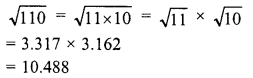Question 20.
1110
Solution: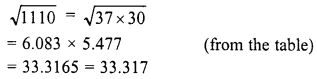Question 21.
11.11
Solution: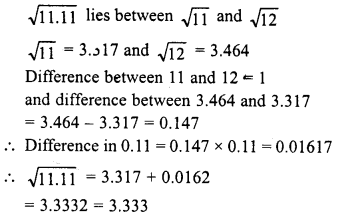Question 22.
The area of a square field is 325 m2. Find the approximate length of one side of the field.
Solution: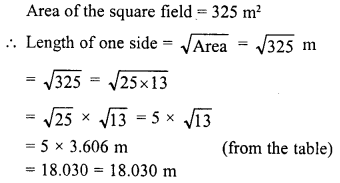Question 23.
Find the length of a side of a square, whose area is equal to the area bf the rectangle with sides 240 m and 70 m.
Solution:All Chapter RD Sharma Solutions For Class 8 Maths

—————————————————————————–

All Subject NCERT Exemplar Problems Solutions For Class 8

All Subject NCERT Solutions For Class 8

*************************************************

I think you got complete solutions for this chapter. If You have any queries regarding this chapter, please comment on the below section our subject teacher will answer you. We tried our best to give complete solutions so you got good marks in your exam.

If these solutions have helped you, you can also share rdsharmasolutions.in to your friends.